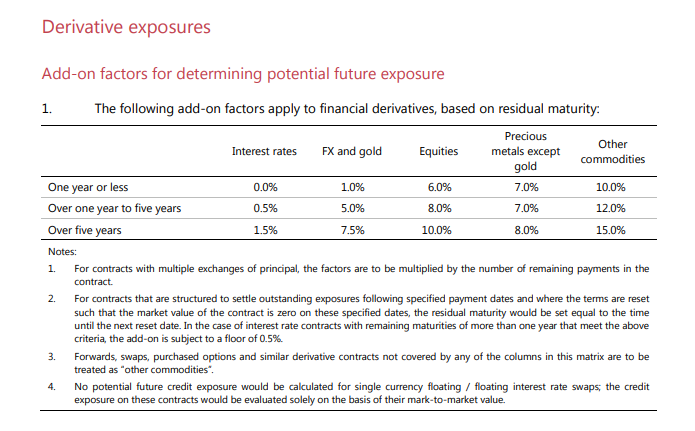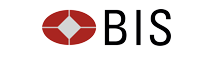# Current Exposure Methodology – What You Need To Know

• The Current Exposure Methodology is a key part of Leverage Ratio calculations.
• It dates back to the late 1980s and the first Basel accords on banking capital.
• CEM calculates the Potential Future Exposure of a derivative trade using a look-up table based on Asset Class and Maturity.
• CEM is a very simple, notional-based measure of derivatives risk.
• PFEs under CEM can be significantly reduced via Compression, improving Leverage Ratios.
• We show you how to calculate PFEs for single trades and portfolios.

### Summary

The Current Exposure Methodology (CEM) can be summarised in a simple look-up table that is used to calculate the Potential Future Exposure of a Derivative contract:But what does this table mean? What are PFEs used for? We examine it through our typical lens of derivatives markets, and in particular the Leverage Ratio. The clearest description for this dates back to January 2014, the BCBS document “Basel III leverage ratio framework and disclosure requirements” or bcbs70.

### Derivatives, Exposures and Notional Amounts

Bank capital is held for a very good reason. If a counterparty defaults on a bilateral loan/derivative/agreement, then a bank should be able to withstand this default without having to unwind all of its’ other obligations. In a trading world, if all counterparties were solely reliant on incoming margin to meet their outgoing margin requirements, then a single default could (would) result in a daisy-chain of defaults.

So, if a bank makes a loan, or if a bank enters a derivative (or even if a bank buys an asset), they must hold a “buffer” against the possibility that this position suddenly no-longer exists.

### Potential Future Exposure of DerivativesWhen a bank makes a loan to a counterparty, it is quite clear what the exposure to the counterparty is – it is the notional amount of the loan. Multiply that by the chances of default, and you have a good idea of how much Capital the bank has to hold against its’ loan book.

But capital also has to be held against derivatives. What is a bank’s exposure to the counterparty at the time of default of a derivative? This is known as the Potential Future Exposure (PFE) of a trade under Basel and it is rarely equal to the full notional of a trade. The Current Exposure Methodology therefore describes how to calculate the PFE in a common way.

### How to Calculate the PFE of a Single Trade

Broadly speaking, to calculate the PFE of a single derivative, we:

• Establish which asset class it belongs to.
• Establish the maturity date of the trade.
• Use the previous look-up table to find the percentage add-on.

For example:

• A \$100m 2 year USD IRS has a PFE of \$500,000.
• A \$100m 6 year EURUSD Cross Currency Swap has a PFE of \$7,500,000.

### How to Calculate the PFE of a Portfolio of Trades

Remember that CEM is a simple way to calculate PFEs for derivatives. It is slightly less straight-forward in how it deals with netting in a portfolio. As Chairman Giancarlo recently highlighted in Swaps Reform 2.0:

CEM’s computation overstates potential exposure dramatically when some positions are long and some are short… CEM attempts to give offsets against this overstated exposure, but the offsets are not strongly risk based and, as a result, range from inadequate to arbitrary.

Netting is a basic concept (e.g. a buy cancels out a sell), but as the Chairman points out, in the trading world it has profound impacts when it is no longer applicable.

Let’s look at the details in CEM – particularly the Net to Gross Ratio.

### Net to Gross Ratio

For the purposes of Leverage Ratio calculations, netting of PFEs is very limited. The documents state that netting should be applied as:Meaning, for a portfolio of trades with a single counterparty, we:

• Calculate the gross PFEs for each trade by referencing its’ asset class and maturity in the PFE look-up table (remember that we ignore trade direction). This is “A_Gross” in the calculation.
• Look at the mark-to-market of each trade (taking into account whether it is positive or negative).
• Calculate the Net to Gross ratio (NGR) of all of these mark-to-markets. i.e. we take the net value and divide it by the sum of the absolute values to calculate a ratio.
• The net replacement cost within this NGR calculation is always floored at zero (it can never be negative).
• We then take 40% of the gross PFEs (“A_Gross”) plus 60% of the gross PFEs (“A_Gross”) multiplied by the NGR (which will be less than 100%, and could be as low as zero if we only owe money to the counterparty).
• Repeat for all other counterparties in the portfolio…..

We could be unfortunate and end up with 100% of our gross PFEs as being our overall portfolio PFE figure. The minimum amount it could ever be is 40% of our gross PFEs. More importantly for derivatives traders, netting is not based on the balance of payers or receivers. Instead, traders are penalised if the portfolio is in-the-money.

### Uses of CEM

For the purposes of capital, CEM is used in two main areas:

1. To measure Credit Risk Weighted Assets (RWAs). However, banks can instead apply for internal model approval to their local regulator to calculate Credit RWAs using their own methodology.
2. For Leverage Ratio exposure calculations (known as the Supplementary Leverage Ratio (SLR) in the US).

### CEM and Leverage Ratio

The Leverage Ratio was introduced to recapitalise the financial system following the financial crisis. It was introduced as an intentionally crude notional-based measure to ensure that more capital was held against derivatives.

Leverage Ratio is particularly punitive to derivative markets:

• The exposure measure for Leverage Ratio (where Leverage Ratio = Capital/Exposure) is calculated using CEM.
• CEM is very sensitive to notional amounts. Interest Rate Derivatives account for huge amounts of notional outstanding.
• Leverage Ratio applies to both new and old trades, cleared and bilateral.
• Leverage Ratio is based upon Gross Notional measures, even at a CCP.

I can’t finish without mentioning Compression. Getting rid of excess notional is hugely beneficial to Leverage Ratios. Following a compression run, gross notional is reduced, therefore requiring less capital to be held versus the same amount of risk. The overall PFE calculation is so sensitive to gross notional, that the effects on the Net to Gross Ratio can be broadly ignored during any compression cycle.

However, our industry never stands still, and changes are afoot in the calculation of Leverage Ratio that could reduce the current focus on compression. We’ll cover this in future blogs.

Stay informed with our FREE newsletter, subscribe here.